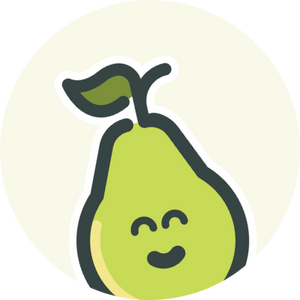Join the Peartember celebration for giveaways, exclusive templates, and more! Learn more >

Engaging Every Student

October 5, 2016

# Layering in MetacognitionPear Deck TeamIn our last post, I wrote about how learning arbitrary things is much harder than learning meaningful things. Culturally, I think we are accepting this fairly broadly — we basically know and believe now that rote memorization isn’t the most effective way to learn.

It’s not just that rote memorization is harder than learning things that are connected, learning in this way also makes it less likely that we will be able to apply our learning or skills to the next thing to be learned. Basically, studies about how we learn show that there is something important about stepping back and considering how we learned. Then, when we face the next new concept, we are better able to use effective learning strategies.

Let’s use our example from last week about introducing supply and demand through the concept of the lemonade stand. Here are some actions a student might do in the course of learning about supply and demand:

• Write down definition of Supply and Demand
• Write down example of Supply and Demand
• Take a test

Now let’s layer in some extra actions that tap into that prior knowledge and make supply and demand relatable:

• Try to figure out how much lemonade they would want to make for a lemonade stand
• Try to figure out how much they would charge for the lemonade
• Try to figure out if this would change if someone opened another stand across the street
• Hear and record definition of Supply and Demand
• Write down how the lemonade stand question are an example of Supply and Demand
• Take a test

Here we see that the student is grappling with the concept before trying to learn the definition. By the time the definition comes along, the student can write down how what they were just figuring out is the practice of supply and demand (instead of writing down an arbitrary example).

Finally, let’s layer in some metacognitive actions a student could do in this scenario.

• Try to figure out how much lemonade they would want to make for a lemonade stand
• Try to figure out how much they would charge for the lemonade
• Write down how did they decided how much to make and charge
• Try to figure out if this would change if someone opened another stand across the street
• Hear and record definition of Supply and Demand
• Write down how the lemonade stand questions are an example of supply and demand
• Write down what things they find confusing about the concept
• Write down questions they have about how the lemonade stand was an example of Supply and Demand